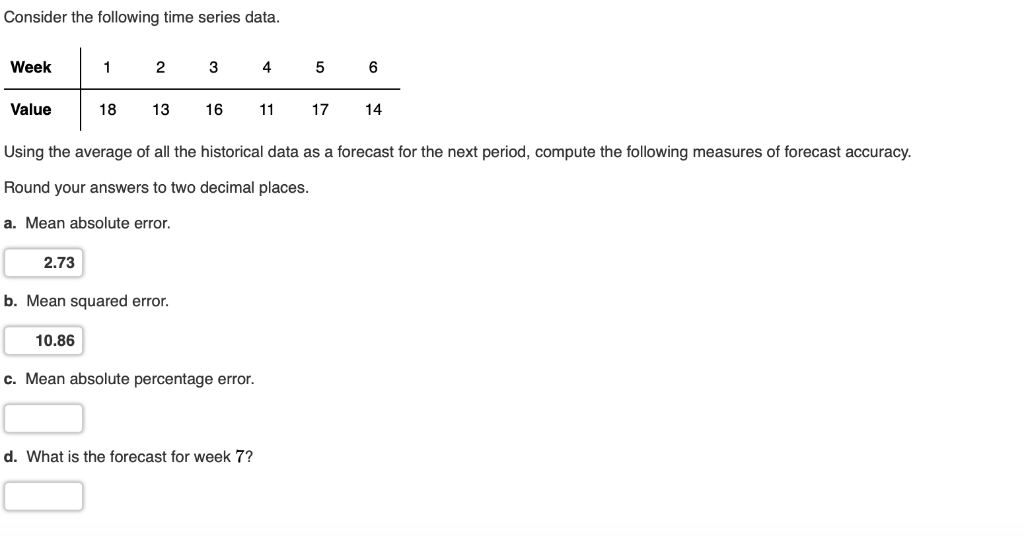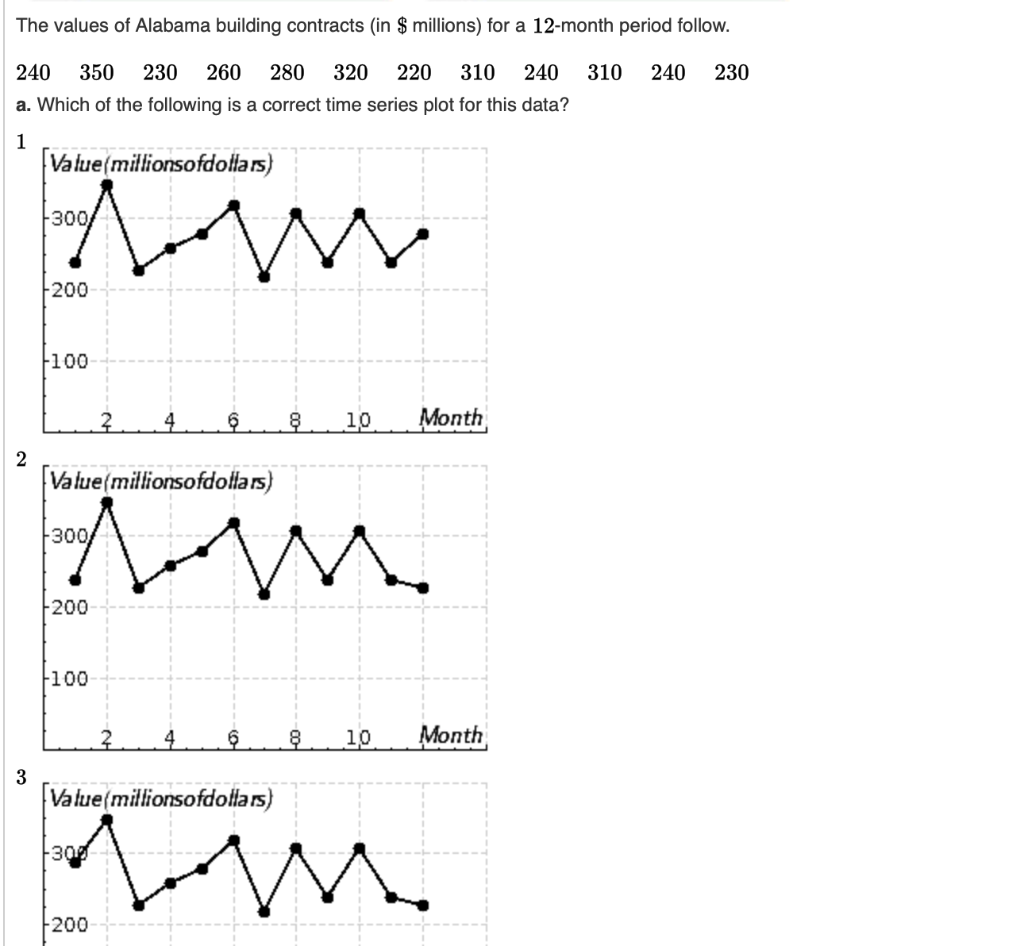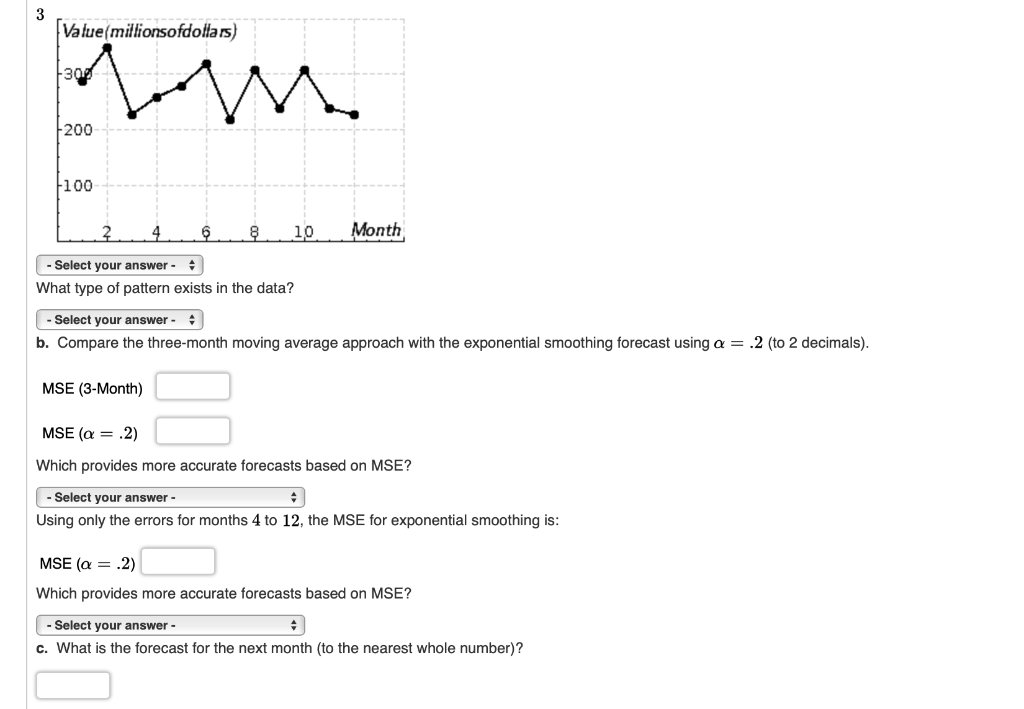# Solved: Consider The Following Time Series Data. Week 1 2 4 5 3 Value 18 13 16 17 14 11 Using The Average Of All The Historical Data As A Forecast For The Next Period, Compute The Following Measures O

By |Consider the following time series data. Week 1 2 4 5 3 Value 18 13 16 17 14 11 Using the average of all the historical data as a forecast for the next period, compute the following measures of forecast accuracy. Round your answers to two decimal places. a. Mean absolute error. 2.73 b. Mean squared error. 10.86 c. Mean absolute percentage error. d. What is the forecast for week 7? The values of Alabama building contracts (in \$ millions) for a 12-month period follow. 240 350 230 260 280 320 220 310 240 310 240 230 a. Which of the following is a correct time series plot for this data? 1 Value(millionsofdollars) 300 200 100 Month 6 10 2 Value(millionsofdollars) 300 200 100 Month 2 4 6 8 10 3 Value(millionsofdollars) 300 200 Value(millionsofdollars) 200 100 Month 4 6 10 Select your answer What type of pattern exists in the data? Select your answer b. Compare the three-month moving average approach with the exponential smoothing forecast using a = .2 (to 2 decimals). MSE (3-Month) MSE (a 2) Which provides more accurate forecasts based on MSE? Select your answer Using only the errors for months 4 to 12, the MSE for exponential smoothing is: MSE (a.2) Which provides more accurate forecasts based on MSE? Select your answer- c. What is the forecast for the next month (to the nearest whole number)?